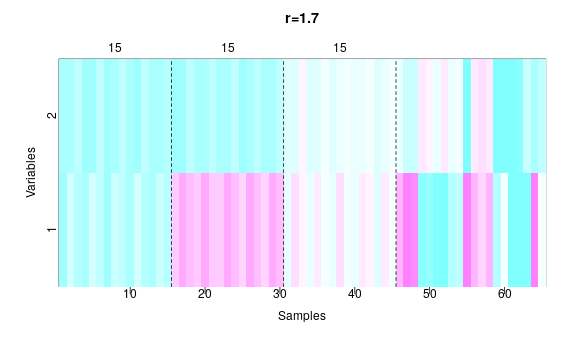# Overview

This package implements the self-updating process clustering algorithm proposed by @Shiu2016supc . This document shows how to reproduce the examples and figures in the paper.

The SUP is a distance-based method for clustering. The idea of this algorithm is similar to gravitational attraction: every sample gravitates towards one another. The algorithm mimics the process of gravitational attraction iteratively that eventually merges the samples into clusters on the sample space. During the iterations, all samples continue moving until the system becomes stable.

We can view this algorithm as standing from the viewpoint of data points to simulate the process how data points move and perform self-clustering. Therefore, this algorithm is named Self-Updating Process(SUP). The user can tune the algorithm by changing the updating rule, which allows for both time-varying and time-invariant operators.

The paper shows that SUP is particularly competitive for:

• Data with noise
• Data with a large number of clusters
• Unbalanced data

# Installation

To install the package from CRAN:

install.packages("supc")


To build the package from source, the Windows user requires Rtools and the Mac OS X user requires gfortran.

To get the current development version from github:

# install.packages('remotes')
remotes::install_github("wush978/supc")


# Algorithm

## Notation

• $$x_1^{(0)}, …, x_N^{(0)} \in \mathbb{R}^{p}$$ are the data to be clustered.
• $$f_t$$ is a function which measures the influence between two data point at the $$t$$-th iteration.
• $$\left\lVert \cdot \right\lVert_p$$ is the $$L_p$$ norm.

## Truncated exponential decay function

Shiu and Chen (2016) showed that a sufficient condition to ensure the convergence of the updating process is that $$f_t$$ is positive and decreasing with respect to distance (PDD). The truncated exponential decay function and the q-Gaussian function are mostly used by the authors. This package only implements $$f_t$$ to be the truncated exponential decay function as presented in the paper.

At the $$t$$-th iteration, the truncated exponential decay function $$f_t$$ is:

$\begin{equation} f_t(x_i^{(t)}, x_j^{(t)}) = \left\{ \begin{array}{lc} exp\left(\cfrac{-\left\lVert x_i^{(t)} - x_j^{(t)} \right\lVert_2}{T(t)}\right) & \text{if } \left\lVert x_i^{(t)} - x_j^{(t)} \right\lVert_2 \leq r \ 0 & \text{if } \left\lVert x_i^{(t)} - x_j^{(t)} \right\lVert_2 > r \end{array} \right. \label{eq:influence} \end{equation}$

## Self-Updating Process

The SUP updates the data points via:

$\begin{equation} x_i^{(t+1)} = \sum_{j=1}^N{\frac{f_t(x_i^{(t)}, x_j^{(t)})}{\sum_{k=1}^N{f_t(x_i^{(t)}, x_k^{(t)})}} x_j^{(t)}} \label{eq:sup} \end{equation}$

The iteration stops after convergence. We compare the $$L_{\infty}$$ distance (i.e. $$max_{1 \leq i \leq N} {\left| x_i^{(t)} - x_i^{(t + 1)} \right|}$$) with tolerance. If the $$L_{\infty}$$ distance is less than tolerance, then the computation stops and returns $$x_1^{(t)}, …, x_N^{(t)}$$.

## Clustering

After retrieving $$x_1^{(t)}, …, x_N^{(t)}$$ from SUP, we compute the connected components of the graph:

$(V, E) = \left(\{1, 2, …, N\}, \left\{ (i,j) | \left\lVert x_i^{(t)} - x_j^{(t)} \right\rVert_2 < tolerance \right\}\right).$

Each connected component is a cluster.

# Usage

The function supc1 in this package implements the SUP clustering.

## Data

The argument x should be a matrix in which the rows represent the samples and the columns represent the variables.

## Parameters

As shown in $$\eqref{eq:influence}$$, SUP requires the following two parameters:

• $$r$$ determines the truncation of the influential function $$f_t$$.
• $$T(t)$$ controls the speed of the convergence.

### Control the parameter $$r$$

supc1 provides two options for users to control the parameter $$r$$.

The user can directly supply the value of $$r$$. If r is given as a vector, then supc1 will compute clustering results using each of the values provided in the numeric vector r. In such a case, supc1 will return a supclist which contains supc objects for each value in r.

Instead of the value of $$r$$, the user can choose to supply the quantile of the pairwise distance between samples. In this case, the user should provide rp, which is a value between 0 and 1. supc1 will compute the pairwise distances between all samples in the data to obtain the $$r$$ value according to the given rp value. If rp is a vector, supc1 will return a supclist, which contains supc objects for each value in rp.

If neither r nor rp is provided, then supc1 will use rp = c(5e-04, 0.001, 0.01, 0.1, 0.3) as the default.

### Control the parameter $$T(t)$$

supc1 has parameter t to control $$T(t)$$, which can be either static or dynamic temperature.

By default, the package uses $$T(t) = \frac{r}{5}$$ and $$T(t) = \frac{r}{20} + t \times \frac{r}{50}$$ for static and dynamic temperature, respectively, which are the temperatures used in the paper. In details, if a character value specified as t = "static", supc1 will set t as function(r) {function(t) {r/5}}, where r is the input value for parameter r. In this case, the temperature is kept as a constant of $$\frac{r}{5}$$ at each iteration. If a character value specified as t = "dynamic", supc1 will set t as function(r)｛function(t) {r/20 + t * (r/50)}}. In this case, the initial temperature is $$\frac{r}{20}$$, and the temperature gradually increases by $$\frac{r}{50}$$ after each iteration.

The package also allows user-specified temperature functions. If t is set as a numeric value, then supc1 uses this value as the static temperature throughout iterations. If t is set as a function, then supc1 will uses the value t(i) as the temperature at each of the $$i$$-th iteration.

The user can use different temperatures $$T(t)$$ with different $$r$$ values by setting t as a list of function. Then supc1 will calculate the clustering result for each pair of $$T(t)$$ and $$r$$.

## Utility

This package allows the user to choose the implementation of computing distances. Currently, stats::dist, amap::Dist and gputools::gpuDist are included. The user could choose the best fitted one according to the data. There is also a c++ implementation of the main algorithm which costs about 50% time compared to the implementation in R.

### Computation of the distance

The user can use dist.mode to select the package to compute the pairwise distances between samples. Specifying dist.mode("stats"), dist.mode("amap") will select stats::dist, amap::Dist to compute the pairwise distance matrix, respectively. Moreover, the user can register function for computing the pairwise distance. For example, one can use GPU to compute the distance via dist.mode("gputools", function(x) gputools::gpuDist(x, method = "euclidean", p = 2.0)) after installing the package gputools from github. It is recommended to compare the computation time of stats::dist(x), amap::Dist(x) or other functions before selecting the function. The default function to compute the pairwise distances is stats::dist.

For example, on my machine whose CPU is Intel(R) Core(TM) i7-4820K CPU @ 3.70GHz and GPU is NVIDIA Corporation GK107GL [Quadro K2000], the computation time of different function is: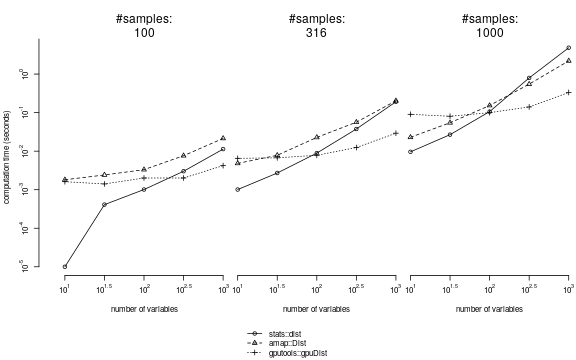The results show that stats::dist is the best choice if the distance matrix is small. gputools::gpuDist outperforms the others if the distance matrix is larger. If there is no GPU, amap::Dist might be a better choice for larger distance matrices.

### Using implementation in R or in C++

This package provides both s in R and in C+++ for better maintenance. The C++ program runs faster when the number of samples is larger. Without specifying, the supc1 will use the implementation in C++ as default. If implementation = "R" is specified, then supc1 will use the implementation in R.

### Verbose

Using supc1 with verbose = TRUE, the program will show the $$L_{\infty}$$ distance between each iteration on the screen in real-time, so that the user can monitor the iterations to check if the program is still running or dead.

## Example

Here we use the following simulated data to demonstrate the use of this package.

### Data

The data of nine small groups is generated. Each group has five normally distributed data points.

set.seed(1)
mu <- list(
x = c(0, 2, 1, 6, 8, 7, 3, 5, 4),
y = c(0, 0, 1, 0, 0, 1, 3, 3, 4)
)
X <- lapply(1:5, function(i) {
cbind(rnorm(9, mu$x, 1/5), rnorm(9, mu$y, 1/5))
})
X <- do.call(rbind, X)


In addition, 20 points representing noise data points are generated to locate 2 units outside from each group center.

n <- nrow(X)
X <- rbind(X, matrix(0, 20, 2))
k <- 1
while(k <= 20) {
tmp <- c(13*runif(1)-2.5, 8*runif(1)-2.5)
y1 <- mu$x - tmp y2 <- mu$y - tmp
y <- sqrt(y1^2+y2^2)
if (min(y)> 2){
X[k+n,] <- tmp
k <- k+1
}
}


The generated data is presented in the following graph, in which noise data points are shown by gray color.

plot(X, col="#999999")
points(X[1:n,])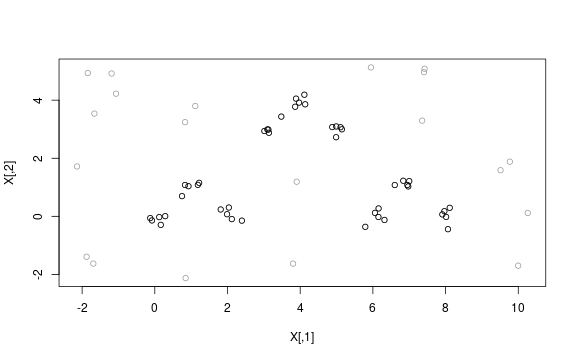### Clustering

The frequency polygon of the pairwise distance may provide useful guideline for the selection of parameter value r. Section 2.4.1 in @Shiu2016supc suggests to use the sharp valleys of the frequency polygon. The user can directly compute the frequency polygon via:

library(supc)
freq.poly(X, breaks = 50)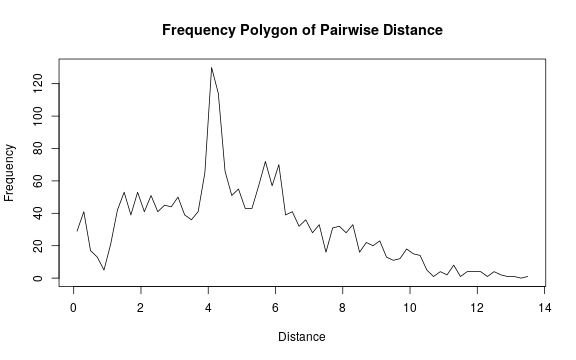The user can also control the number of bins by specifying the argument breaks. Please see ?hist for details.

The object returned by freq.poly is the same as the object returned by the hist function. In this example, X.freq$mids is the vector of x-axis and X.freq$count is the vector of y-axis of the frequency plot. The user can use these two vectors to identify the exact locations of the valleys of the polygon.

After examing the data, there are sharp valleys at 0.9, 1.7, 2.1, 2.3, and more.

X.freq <- freq.poly(X, breaks = 50)
abline(v = c(0.9, 1.7, 2.1, 2.5), lty = 2)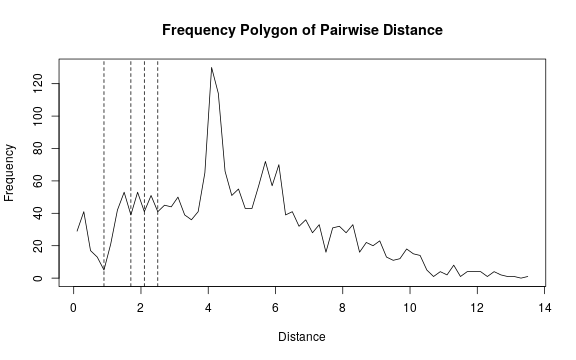For demonstration, the following computes clustering result by SUP using supc::supc1 with r = 1.7 and t = "static" (that is $$T = r/5$$).

library(supc)
X.supc <- supc1(X, r = 1.7, t = "static")


The returned object has class “supc” which contains:

str(X.supc)

## List of 9
##  $x : num [1:65, 1:2] -0.125 2.037 0.833 6.319 8.066 ... ##$ d0       : NULL
##  $r : num 1.7 ##$ t        :function (t)
##  $cluster : int [1:65] 1 1 1 2 2 2 3 3 3 1 ... ##$ centers  : num [1:13, 1:2] 1.06 6.91 4.01 7.11 9.78 ...
##   ..- attr(*, "dimnames")=List of 2
##   .. ..$: chr [1:13] "1" "2" "3" "4" ... ## .. ..$ : NULL
##  $size : 'table' int [1:13(1d)] 15 15 15 4 3 4 2 1 1 1 ... ## ..- attr(*, "dimnames")=List of 1 ## .. ..$ cl: chr [1:13] "1" "2" "3" "4" ...
##  $result : num [1:65, 1:2] 1.06 1.06 1.06 6.91 6.91 ... ##$ iteration: int 25
##  - attr(*, "class")= chr "supc"

• x, the input data.
• d0, the distance matrix of the data.
• r, the value of $$r$$.
• t, the function of $$T(t)$$.
• cluster, the cluster label of each sample.
• centers, the center of each cluster.
• size, the size of each cluster.

#### Cluster

We could retrieve the cluster ID of the samples via:

X.supc$cluster  ##  1 1 1 2 2 2 3 3 3 1 1 1 2 2 2 3 3 3 1 1 1 2 2 ##  2 3 3 3 1 1 1 2 2 2 3 3 3 1 1 1 2 2 2 3 3 3 4 ##  5 6 6 7 8 4 4 5 9 4 10 7 6 11 12 11 5 6 13  This vector represents the IDs of the resulting clusters of each sample. For example, the first sample, i.e. X[1,], is clustered into cluster ID X.supc$cluster.

#### Centers

The centers of the resulting clusters are stored in X.supc$centers, which are the converged locations of data points after iterations: X.supc$centers

##          [,1]       [,2]
## 1   1.0605950  0.3482244
## 2   6.9065178  0.4280574
## 3   4.0071623  3.3813771
## 4   7.1095628  4.8002607
## 5   9.7824613  1.3620980
## 6  -1.4223446  4.4789023
## 7   0.9731686  3.5219031
## 8   9.9983292 -1.6988732
## 9   0.8490764 -2.1283129
## 10  3.8048658 -1.6295495
## 11 -1.7838743 -1.5093699
## 12 -2.1387674  1.7184862
## 13  3.9037496  1.1924147


X.supc$size  ## cl ## 1 2 3 4 5 6 7 8 9 10 11 12 13 ## 15 15 15 4 3 4 2 1 1 1 2 1 1  The cluster IDs are ordered by cluster size. Note that this clustering result shows that SUP identified 13 clusters. The three major clusters are consisted of data points from nine normally distributed groups. The rest 10 clusters are identified to be consisted of noise data points. ### supclist To compute clustering results by multiple parameters, the user can directly pass a vector to the corresponding parameters r, rp, or t. For demonstration, the following uses r = c(0.9, 1.7, 2.5) according to the approximate locations of sharp values in the frequency polygon of pairwise distances. X.supcs <- supc1(X, r = c(0.9, 1.7, 2.5), t = "dynamic")  The supc1 will compute the results of all given r values and t values. In this case, the X.supcs is a supclist object. The user can retrieve all cluster IDs via: X.supcs$cluster

## $r=0.900000 ##  1 2 3 4 5 6 7 8 9 1 2 3 4 5 6 7 8 9 1 2 3 4 5 ##  6 7 8 9 1 2 3 4 5 6 7 8 9 1 2 3 4 5 6 7 8 9 10 ##  11 12 12 13 14 15 16 11 17 15 18 13 19 20 21 20 22 12 23 ## ##$r=1.700000
##    1  1  1  2  2  2  3  3  3  1  1  1  2  2  2  3  3  3  1  1  1  2  2
##   2  3  3  3  1  1  1  2  2  2  3  3  3  1  1  1  2  2  2  3  3  3  4
##   5  6  6  7  8  9  9  5 10  9 11  7  6 12 13 12 14  6 15
##
## \$r=2.500000
##   1 1 1 2 2 2 3 3 3 1 1 1 2 2 2 3 3 3 1 1 1 2 2 2 3 3 3 1 1 1 2 2 2 3 3
##  3 1 1 1 2 2 2 3 3 3 4 2 5 5 6 2 4 4 2 1 4 7 6 5 8 9 8 2 5 3


The following graphs show clustering results using different r values. The IDs of the clusters each sample is clustered into is plotted in the graph. The major clusters are marked by blue circles. Note that the size of the major clusters increases with r value.

The results show that the use of r = 0.9 produces 23 clusters, including nine major clusters and 14 tiny clusters that can be identified as noise. The use of r = 1.7 produces 15 clusters, including three major clusters and 12 tiny clusters that can be identified as noise. When the influential range r is set as large as 2.5, 9 clusters are identified. The three major clusters consist of mixed data from both the normally distributed data points and the noise data points.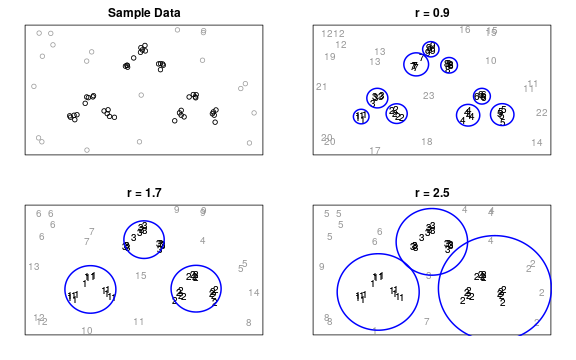### Heatmap

The user can use heatmaps to further understand the structure of the data and the patterns within each cluster.

The following graphs show the heatmaps of the data using clustering results with different r values.

plot(X.supcs[], type = "heatmap", major.size = 2)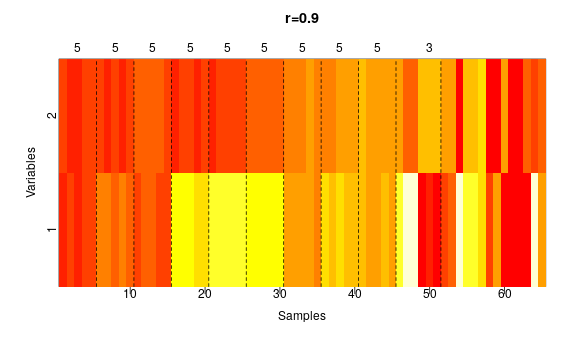After specifying type = "heatmap", the plot function will draw the corresponding heatmap of the given supc object. The samples will be ordered by the cluster IDs. The dashed lines split the samples into different clusters and the cluster size is annotated above the figure. For those clusters whose size is smaller than the parameter major.size, the dashed lines will be omitted.

The user could pass additional parameters to the underlying image function. For example:

plot(X.supcs[], type = "heatmap", col = cm.colors(24), major.size = 5)